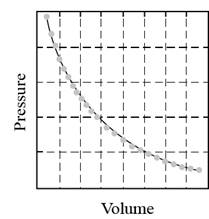Chapter 15, Problem 5CR### Introductory Chemistry: A Foundati...

9th Edition
Steven S. Zumdahl + 1 other
ISBN: 9781337399425

#### Solutions

Chapter
Section### Introductory Chemistry: A Foundati...

9th Edition
Steven S. Zumdahl + 1 other
ISBN: 9781337399425
Textbook Problem
1 views

# m>5. When using Boyle’s law in solving problems in the textbook, you may have noticed that questions were often qualified by stating that the temperature and amount of gas remain the same.” Why was this qualification necessary?

Interpretation Introduction

Interpretation:

The reason as to why the qualification is necessary in stating the statement “the temperature and amount of gas remain the same” is to be stated.

Concept Introduction:

Boyle’s law states that if the temperature and the moles of gas remain constant, then the pressure exerted by the gas is inversely proportional to the volume occupied by the gas in that space.

The mathematical statement of Boyle’s law is,

Pα1V

Or

PV=constant

The Boyle’s law can also be expressed graphically as:Figure 1.

Explanation

According to the law, pressure is inversely proportional to volume. The volume of a gas also depepnds on other factors like temperature (Charle’s law) and number of moles (Avogadro’s law). Hence, while stating the relationship between two pa...

### Still sussing out bartleby?

Check out a sample textbook solution.

See a sample solution

#### The Solution to Your Study Problems

Bartleby provides explanations to thousands of textbook problems written by our experts, many with advanced degrees!

Get Started